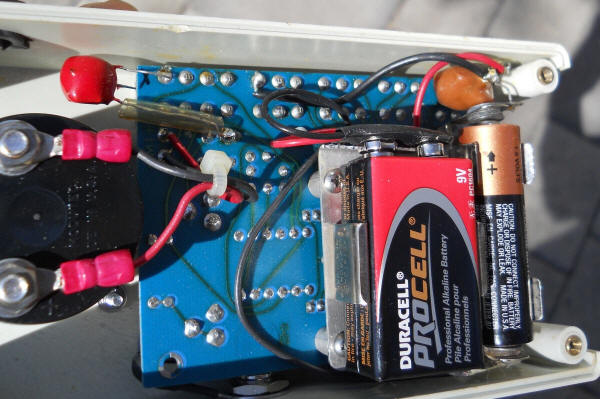# Mercury Battery Replacement

There are many older battery-powered instruments on the surplus market that require mercury batteries but these batteries have been banned for environmental reasons. Simply substituting another battery type may give unsatisfactory results; mercury batteries have an unusually stable output voltage over their life and many designs rely on this stability for proper operation.

The following circuit simulates a single mercury battery cell (1.35 volts) for low current loads and is powered by a couple of ordinary cells. (Two, 1.5 volt alkaline cells in series would be a typical power source.) The circuit automatically turns on when power is demanded so no power switch is necessary.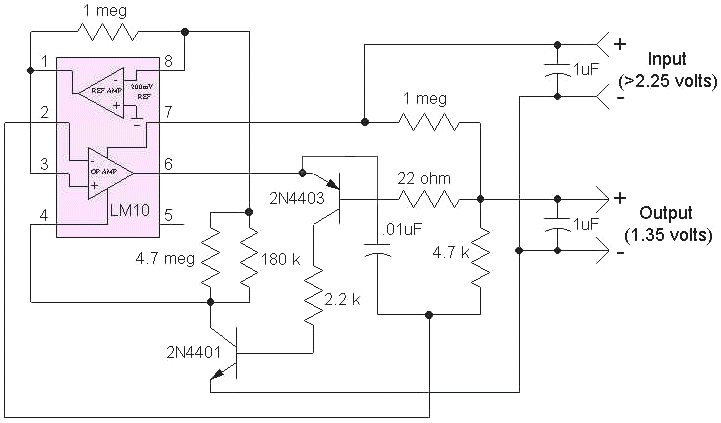The reference portion of the LM10 supplies the desired output voltage to the op-amp section and this voltage may be adjusted by changing the 4.7 meg and 180k in the collector of the 2N4401. The output current flows through the emitter-base junction of the 2N4403, turning it and the 2N4401 on, activating the regulator. The 22 ohm, .01 uF and 4.7k help to stabilize the circuit for large capacitive loads. If the load is resistive, those three components may be replaced with a short but check for oscillations on the output. The 22 ohm may be reduced for higher current loads but below about 10 ohms, stability may become a problem if the load includes a large shunt capacitor. Increasing the input voltage will increase the available current but the LM10 will limit the ultimate current to about 20 mA so this circuit will not drive motors!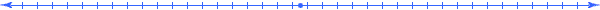Also consider a shunt regulator when you can get to the other side of the power switch. The following circuit was used to supply about 500uA to a pH meter/simulator. Notice how the output of the op-amp is connected directly to the power pin. When the voltage across the op-amp exceeds 1.35 volts, the output stage sinks current, lowering the voltage. The 100 ohm resistor determines how much current is consumed.  Determine how much current your circuit needs then select this value to supply the required current with about .05 volts across it (R = .05/I). For my 500uA load and with a fresh battery at about 1.6 volts, the voltage across the 100 ohm resistor is 1.6 - 1.35 = .25 volts and the current is about 2.5 mA, easily handled by an alkaline AA cell. The voltage on the battery can drop down to 1.4 volts before regulation is lost. For a higher current load or a lower drop-out voltage, lower the 100 ohm resistor value. The 57.5 k resistor was selected to achieve exactly 1.35 volts and consisted of two resistors in parallel.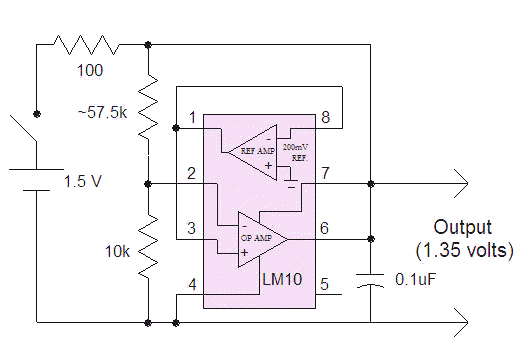This circuit can only supply low currents, too, since the LM10 must shunt whatever is not used by the load and it can only sink about 20 mA. So the load current can only change by 20 mA, max. That is fine for most gadgets but some devices might have momentary current demands in excess of that amount (especially if a motor is involved). One could add a super-cap across the output of these regulators to supply high transient currents.For higher voltage situations, consider a simple voltage regulator: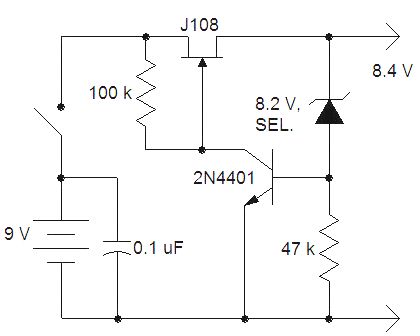The zener is selected to give the desired 8.4 volts output. The J108 can supply a few mA with virtually no voltage drop so this circuit will work in many mercury battery applications until the 9 volt battery drops below 8.4 volts. It is true that 8.4 volts is a little soon to be tossing a 9 volt battery. A 1.5 volt AAA cell could be added in series with the 9 volt battery to extend the life of the battery but a fresh alkaline will serve well for most of its useful life; just put the depleted battery in a radio or voltmeter to get the last bit of life. Keep in mind that in many of the devices that use a couple of mercury batteries, the higher voltage battery does not need to exhibit a precise voltage. My pH meter/simulator relies on the precision of the 1.35 volt battery, for example and the 8.4 volt battery simply powers an op-amp. This circuit loses regulation gracefully and the output voltage simply equals the battery voltage below 8.4 volts.

Here's the view in the pH meter/simulator. The red blob below drops the 9 volts to 8.4 volts and the orange blob drops the 1.5 volts to 1.35 volts.